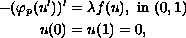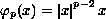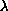Electron. J. Diff. Eqns., Vol. 2000(2000), No. 52, pp. 1-42.

### Multiplicity results for classes of one-dimensional p-Laplacian boundary-value problems with cubic-like nonlinearities Idris Addou

Abstract:
We study boundary-value problems of the typewhere p>1,, andis positive. We provide multiplicity results when f behaves like a cubic with three distinct roots, at which it satisfies Lipschitz-type conditions involving a parameter q>1. We shall show how changes in the position of q with respect to p lead to different behavior of the solution set. When dealing with sign-changing solutions, we assume that f is half-odd; a condition generalizing the usual oddness. We use a quadrature method.

Submitted April 16, 1999. Revised May 1, 2000. Published July 3, 2000.
Math Subject Classifications: 34B15.
Key Words: p-Laplacian, time-maps, multiplicity results, cubic-like nonlinearities.

Show me the PDF file (312K), TEX file, and other files for this article.Idris Addou USTHB, Institut de Mathematiques El-Alia, B.P. no. 32 Bab-Ezzouar 16111, Alger, Algerie. e-mail: idrisaddou@hotmail.com